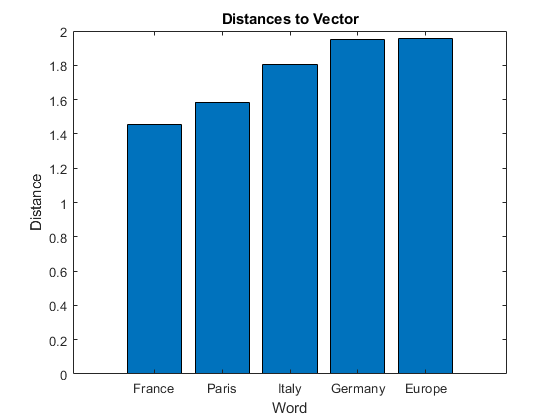# vec2word

Map embedding vector to word

## Syntax

``words = vec2word(emb,M)``
``[words,dist] = vec2word(emb,M)``
``___ = vec2word(emb,M,k)``
``___ = vec2word(___,'Distance',distance)``

## Description

example

````words = vec2word(emb,M)` returns the closest words to the embedding vectors in the rows of `M`.```

example

````[words,dist] = vec2word(emb,M)` returns the closest words to the embedding vectors in `M`, and returns the distances `dist` of each to their source vectors.```

example

````___ = vec2word(emb,M,k)` returns the top `k` closest words.```

example

````___ = vec2word(___,'Distance',distance)` specifies the distance metric.```

## Examples

collapse all

Load a pretrained word embedding using `fastTextWordEmbedding`. This function requires Text Analytics Toolbox™ Model for fastText English 16 Billion Token Word Embedding support package. If this support package is not installed, then the function provides a download link.

`emb = fastTextWordEmbedding`
```emb = wordEmbedding with properties: Dimension: 300 Vocabulary: [1×1000000 string] ```

Map the words "Italy", "Rome", and "Paris" to vectors using `word2vec`.

```italy = word2vec(emb,"Italy"); rome = word2vec(emb,"Rome"); paris = word2vec(emb,"Paris");```

Map the vector `italy - rome + paris` to a word using `vec2word`.

`word = vec2word(emb,italy - rome + paris)`
```word = "France" ```

Find the top five closest words to a word embedding vector and their distances.

Load a pretrained word embedding using `fastTextWordEmbedding`. This function requires Text Analytics Toolbox™ Model for fastText English 16 Billion Token Word Embedding support package. If this support package is not installed, then the function provides a download link.

`emb = fastTextWordEmbedding;`

Map the words "Italy", "Rome", and "Paris" to vectors using word2vec.

```italy = word2vec(emb,"Italy"); rome = word2vec(emb,"Rome"); paris = word2vec(emb,"Paris");```

Map the vector `italy - rome + paris` to a word using `vec2word`. Find the top five closest words using the Euclidean distance metric.

```k = 5; M = italy - rome + paris; [words,dist] = vec2word(emb,M,k,'Distance','euclidean');```

Plot the words and distances in a bar chart.

```figure; bar(dist) xticklabels(words) xlabel("Word") ylabel("Distance") title("Distances to Vector")```## Input Arguments

collapse all

Input word embedding, specified as a `wordEmbedding` object.

Word embedding vectors, specified as a matrix. Each row of `M` is a word embedding vector. `M` must have `emb.Dimension` columns.

Number of closest words to return, specified as a positive integer.

Distance metric, specified as `'cosine'` or `'euclidean'`.

## Output Arguments

collapse all

Output words, returned as a string vector.

Distance of words to their source vectors, returned as a vector.

Introduced in R2017b